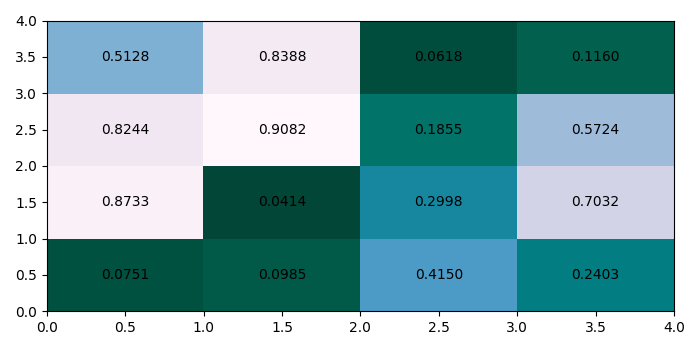# How to annotate a heatmap with text in Matplotlib?

To annotate a heatmap with text in matplotlib, we can take the following steps −

• Create random data with 4×4 dimension array.

• Create a pseudocolor plot with a non-regular rectangular grid, using pcolor() method.

• To put text in the pixels, we can use text() method.

• To display the figure, use show() method.

## Example

import matplotlib.pyplot as plt
import numpy as np
plt.rcParams["figure.figsize"] = [7.00, 3.50]
plt.rcParams["figure.autolayout"] = True
data = np.random.rand(4, 4)
heatmap = plt.pcolor(data, cmap="PuBuGn_r")
for y in range(data.shape):
for x in range(data.shape):
plt.text(x + 0.5, y + 0.5, '%.4f' % data[y, x],
horizontalalignment='center',
verticalalignment='center',
)
plt.show()

## Output